2022: SklogWiki celebrates 15 years on-line

# Difference between revisions of "Stirling's approximation"

James Stirling (1692-1770, Scotland)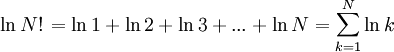$\left.\ln N!\right. = \ln 1 + \ln 2 + \ln 3 + ... + \ln N = \sum_{k=1}^N \ln k$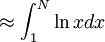$~\approx \int_1^N \ln x dx$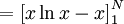$~= \left[ x \ln x - x \right]_1^N$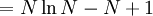$~= N \ln N -N +1$

Thus, for large N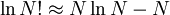$\ln N! \approx N \ln N -N$